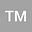Asymptotic analysis of spectral problems in thick junctions with the branched fractal structure
•## Abstract

A spectral problem is considered in a domain $\Omega_{\varepsilon}$ that is the union of a domain $\Omega_{0}$ and a lot of thin trees situated $\varepsilon$-periodically along some manifold on the boundary of $\Omega_{0}.$ The trees have finite number of branching levels. The perturbed Robin boundary condition $\partial_{\nu}u^{\varepsilon} + \varepsilon^{\alpha_i} k_{i,m}u^{\varepsilon} = 0$ is given on the $i$th branching layer; $\{\alpha_i\}$ are real parameters. The asymptotic analysis of this problem is made as $\varepsilon\to0,$ i.e., when the number of the thin trees infinitely increases and their thickness vanishes. In particular, the Hausdorff convergence of the spectrum to the spectrum of the corresponding nonstandard homogenized spectral problem is proved, the leading terms of asymptotics are constructed, and the corresponding asymptotic estimates are justified for the eigenvalues and eigenfunctions.
10 Feb 2022Submitted to Mathematical Methods in the Applied Sciences
12 Feb 2022Submission Checks Completed
12 Feb 2022Assigned to Editor
29 Mar 2022Reviewer(s) Assigned
06 May 2022Review(s) Completed, Editorial Evaluation Pending
11 May 2022Editorial Decision: Revise Minor